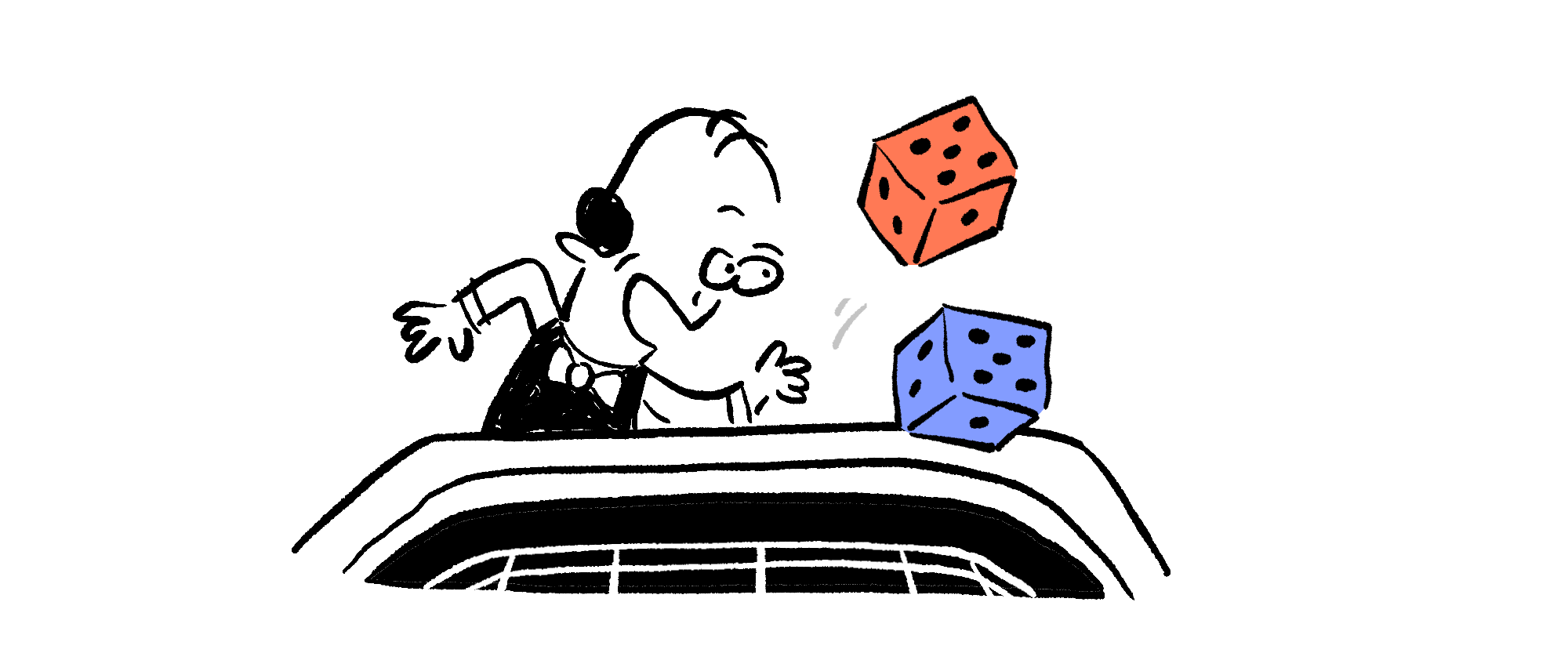Back

## A Winning Combination

Let's say you're walking along, minding your own business, when a stranger asks if you'd like to play a game. Being very bored, you agree. The stranger explains the rules, saying that you will roll a pair of fair, standard six-sided dice. If you roll a sum of seven, you win. If you roll a sum of any other number, you lose.It doesn't seem that the odds are in your favor, but we can describe exactly how likely it is that you win with a probability. Keep reading for some insight on how we might do this, or skip ahead to the challenge if you feel ready to try it on your own.

It might seem that there's only one way to win (roll a total of seven), but there's more than one way to do that. Let's first focus on counting those ways.

If you roll a one with the blue die, rolling a six on the red die gives you a sum of seven. Similarly, a two on the blue die and a five on the red die also gives you a total of seven. There are six ways to roll a sum of seven:

$\def\arraystretch{1.5}\begin{array}{l|cccccc} \color{#3D99F6}\text{Blue Die} & 1 & 2 & 3 & 4 & 5 & 6 \\ \color{#D61F06}\text{Red Die} & 6 & 5 & 4 & 3 & 2 & 1 \end{array}$

We've counted all the winning rolls; how many rolls are there in all?

Each die has six possible numbers that might come up. When trying to count how many possibilities that gives us, it might help to assume one of the dice has had a specific number come up. For example, let's say you've rolled a one with the blue die, as we did when we started counting winning rolls. The red die could then have any of its six numbers show up. That gives us six rolls where the blue die has a one:

$\def\arraystretch{1.5}\begin{array}{l|cccccc} \color{#3D99F6}\text{Blue Die} & 1 & 1 & 1 & 1 & 1 & 1 \\ \color{#D61F06}\text{Red Die} & 1 & 2 & 3 & 4 & 5 & 6 \end{array}$

Similarly, there are six rolls where the blue die comes up with a two on top:

$\def\arraystretch{1.5}\begin{array}{l|cccccc} \color{#3D99F6}\text{Blue Die} & 2 & 2 & 2 & 2 & 2 & 2 \\ \color{#D61F06}\text{Red Die} & 1 & 2 & 3 & 4 & 5 & 6 \end{array}$

There are six possible numbers the blue die can have, so there are $6 \times 6 = \mathbf{36}$ possible rolls in all.

The dice are fair, which means every possible roll has the same chance of coming up. How does that let you find the probability that you win?

# Today's Challenge

You are playing a game where you roll a pair of fair, standard six-sided dice. If you roll a sum of seven, you win. If you do not, you lose.Are you more likely to win by rolling two dice at the same time, or by rolling a single die two times?×